# Print all the even nodes of a Binary Search Tree

## Introduction

### Binary search tree (BST)

Before jumping to the question, let’s understand what a Binary search tree is:

A Binary search tree (BST)  is a type of binary tree in which the nodes are arranged in a specific order. It is also known as an ordered binary tree.

Properties of a Binary search tree (BST):

• The value of all the nodes in the left subtree of a node is smaller than the node’s value.
• All the nodes in the right subtree of a node are greater than the node’s value.
• All the root node’s left and right subtrees must follow the above properties.

Example: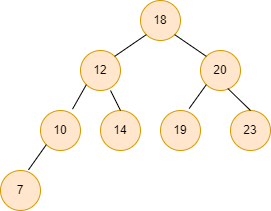### Problem statement

We are given a Binary search tree. Our task is to print all the even nodes (nodes with even values) of the given Binary search tree.

Example:

Input1: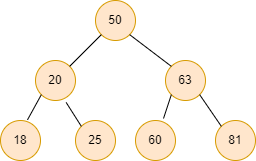Output1: 18 20 50 60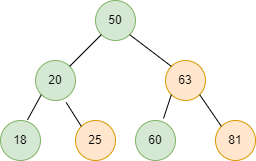Input2: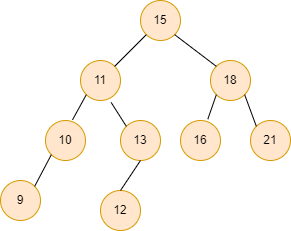Output2: 10 12 16 18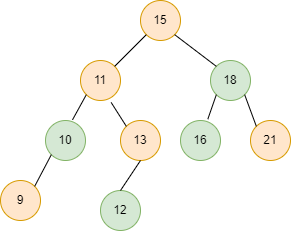## Approach

The idea is straightforward: traverse all the Binary search tree nodes. We check for every node if the current node’s value is even or not. If the current node’s value is even, we print the node. Else we ignore the node.

For traversing the tree, we can use any traversal technique. Here we are using the inorder traversal (left root right) technique for traversing the Binary search tree because it prints all the even nodes of the Binary search tree from left to right order.

Recursive code of inorder traversal:

We can modify the above code of the inorder traversal technique to print only the even nodes of the Binary search tree. So, we add that if the current node’s value is even, then only print the node else, ignore the node, traverse the other nodes, and do the same process.

Steps of print_even_nodes() function:

• Recursively call the print_even_nodes() function for the left subtree.
• Print the root->data if the root value is even.
• Recursively call the print_even_nodes() function for the right subtree.

## Code in C++

``````#include <bits/stdc++.h>
using namespace std;

struct Node {
int data;
struct Node* left;
struct Node* right;
};

Node* new_node(int x)
{
Node* freshnode = new Node();
freshnode->data = x;
freshnode->left = NULL;
freshnode->right = NULL;

return (freshnode);
}
Node* insert_bst( Node* node, int data)
{

if (node == NULL)
return (new_node(data));
else {
if (data <= node->data)
node->left = insert_bst(node->left, data);
else
node->right = insert_bst(node->right, data);

return node;
}
}

void print_even_nodes( Node* root)
{
if (root == NULL)
return;
print_even_nodes(root->left);
if (root->data % 2 == 0)
cout << root->data << " ";
print_even_nodes(root->right);
}

int main()
{
Node* root = NULL;
root = insert_bst(root, 50);
insert_bst(root, 20);
insert_bst(root, 63);
insert_bst(root, 18);
insert_bst(root, 25);
insert_bst(root, 60);
insert_bst(root, 81);

print_even_nodes(root);

cout << endl;

return 0;
}``````

Output

``18 20 50 60``

### Complexity Analysis

Time complexity: O(n), where n is the number of nodes in the given tree, traversing all the nodes in the Binary search tree.

Space complexity: O(n), as the recursion stack at max contains all the nodes of the Binary search tree.

Q1. How does a binary search tree work?

Ans: The Binary search tree works so that each element that is to be inserted is sorted as soon as it is inserted. The comparison begins at the root and proceeds to the left or right sub-trees, depending on whether the value to be inserted is less than or greater than the root.

Q2. In a binary search tree, how do you add and remove elements?

Ans: Step1: Compare the value inserted with the current node value at the Tree's root node.

Step2: Go to the left subtree if the value to be inserted is less than or equal to the root node value; otherwise, go to the right subtree.

Step3: Compare the value to the value of the subtree's root node, and then repeat step 2.

Q3. Are binary trees valuable?

Ans: Binary trees are primarily used in computing for searching and sorting because they allow data to be stored hierarchically. Insertion, deletion, and traversal are the most common operations performed on binary trees.

## Key Takeaways

So, this article discussed the binary search tree, its properties and the approach of printing all the even nodes (node with even values) of the Binary search tree by modifying the inorder traversal code with its complete explanation and code in C++.

If you are a beginner, interested in coding and want to learn DSA, you can look for our guided path for DSA, which is free!